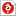검색어 입력폼

# 실험2(결과)

저작시기 2010.10 |등록일 2012.01.02한컴오피스 (hwp) | 13페이지 | 가격 1,000원

## 소개글

Spectral Analysis(결과)

없음

## 본문내용

■ Unit Test
1. What does the FREQUENCY SPAN control refer to, on the Spectrum Analyzer?a. To the maximum length of the horizontal scale;b. To the maximum height of the vertical scale;ⓒ. To the frequency difference equivalent to one horizontal division;d. To the frequency difference equivalent to one vertical division;
2. What is meant by LOG SCALE 10 dB/V?a. Each 1 V horizontal division represents 10 dB;b. Each 1 V vertical division represents 10 dB;c. Each 1 V horizontal and vertical division represents 10 dB;ⓓ. Each 1 V vertical division represents 10 dB if the OUTPUT LEVEL control is in the CAL position.
3. The MAXIMUM INPUT indicatesa. that this level must not be exceeded;b. that each division on the screen represents this level;c. that each vertical division on the screen represents this level;d. that 6 vertical divisions correspond to this level;ⓔ. both a and d.
4. Spectral analysis of a signal provides relative information abouta. amplitude changes with respect to time;b. phase changes with respect to time;ⓒ. the harmonics of a signal;d. both b and c.
5. Spectral analysis can provide information abouta. the phase of harmonics;b. phase changes with respect to time;ⓒ. the harmonics of a signal;d. both a and b.
6. The MARKERS on the Spectrum Analyzer can be used for precise measurements of:ⓐ. frequency;b. amplitude;c. both a and b;d. none of the above.

## 참고 자료

http://www.tpub.com/content/neets/14181/